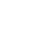# 空间搜索原理

1）对Point（经纬度）和其他的几何图形建索引

2）根据距离排序

3）根据矩形，圆形或者其他的几何形状过滤搜索结果

###GeoHash算法

####根据经纬度计算GeoHash二进制编码

1）区间[-90,90]进行二分为[-90,0),[0,90]，称为左右区间，可以确定39.92324属于右区间[0,90]，给标记为1；

2）接着将区间[0,90]进行二分为 [0,45),[45,90]，可以确定39.92324属于左区间 [0,45)，给标记为0；

3）递归上述过程39.92324总是属于某个区间[a,b]。随着每次迭代区间[a,b]总在缩小，并越来越逼近39.928167；

4）如果给定的纬度（39.92324）属于左区间，则记录0，如果属于右区间则记录1，这样随着算法的进行会产生一个序列1011 1000 1100 0111 1001，序列的长度跟给定的区间划分次数有关。####组码###Cartesian Tiers 笛卡尔层1、根据Cartesian Tier层获得坐标点的地理位置gridId
2、与系统索引gridId匹配计算
3、计算结果集与目标坐标点的距离返回特定范围内的结果集合

# 基于Solr的空间搜索实践

1）索引的构建

2）查询

###索引构建

####Step1 定义空间索引类型和字段

``````<fieldType  name="location_rpt"     class="solr.SpatialRecursivePrefixTreeFieldType" spatialContextFactory="com.spatial4j.core.context.jts.JtsSpatialContextFactory" distErrPct="0.025" maxDistErr="0.000009" units="degrees"/>

<field name=”poi_location_p"  type="location_rpt"  indexed="true"     stored="true"     multiValued=”false"/>
``````

SpatialRecursivePrefixTreeFieldType

JtsSpatialContextFactory

distErrPct

geo

worldBounds

distCalculator

prefixTree

maxDistErr/maxLevels

maxDistErr定义了索引数据的最高层maxLevels，上述定义为0.000009，根据GeohashUtils.lookupHashLenForWidthHeight(0.000009, 0.000009)算出编码长度为11位，精度在1米左右，直接决定了Point索引的term数。
maxLevels优先级高于maxDistErr,即有maxLevels的话maxDistErr失效。详见SpatialPrefixTreeFactory.init()方法。

units

####Step2 构建索引

``````doc.setField(”poi_location_p",     "32.52162,120.31778") //point类型

doc.setField("poi_location_p",     "POLYGON((120.35330414772034
31.58268495951037,120.35190939903259     31.57923921490961,120.35330414772034
31.58268495951037))") //多边形类型
``````2、创建索引域,具体过程参考org.apache.lucene.spatial.prefix.RecursivePrefixTreeStrategy中的createIndexableFields方法。

a、根据distErrPct字段，计算距离的误差值，对于Point来说默认为0（而对于非Point类型来说，是通过外包矩形中心点到矩形顶点的距离再乘以distErrPct来计算误差值的）

``````double distErr = SpatialArgs.calcDistanceFromErrPct(shape, distErrPct, ctx);

public static double calcDistanceFromErrPct(Shape shape, double distErrPct, SpatialContext ctx) {
if (distErrPct < 0 || distErrPct > 0.5) {
throw new IllegalArgumentException("distErrPct " + distErrPct + " must be between [0 to 0.5]");
}
if (distErrPct == 0 || shape instanceof Point) {
return 0;
}
Rectangle bbox = shape.getBoundingBox();
//Compute the distance from the center to a corner.  Because the distance
// to a bottom corner vs a top corner can vary in a geospatial scenario,
// take the closest one (greater precision).
Point ctr = bbox.getCenter();
double y = (ctr.getY() >= 0 ? bbox.getMaxY() : bbox.getMinY());
double diagonalDist = ctx.getDistCalc().distance(ctr, bbox.getMaxX(), y);
return diagonalDist * distErrPct;
}
``````

b、根据上述计算出的误差值，得到索引的geohash编码长度，对于Point类型来说值为maxLevels。

``````public int getLevelForDistance(double dist) {
if (dist == 0)
return maxLevels;//short circuit
final int level = GeohashUtils.lookupHashLenForWidthHeight(dist, dist);
return Math.max(Math.min(level, maxLevels), 1);
}
``````

c、根据编码长度得到满足所有条件的cells（每个cell表示一个前缀值），并将Cells都放在CellTokenStream中，同时构建索引域。Point类型每个Cell表示geohash的一个前缀值。

``````public List<Cell> getCells(Point p, int detailLevel, boolean inclParents){
Cell cell = getCell(p, detailLevel);
if (!inclParents) {
return Collections.singletonList(cell);
}

String endToken = cell.getTokenString();
assert endToken.length() == detailLevel;
List<Cell> cells = new ArrayList<Cell>(detailLevel);
for (int i = 1; i < detailLevel; i++) {
}
return cells;
}

Field field = new Field(getFieldName(),
new CellTokenStream(cells.iterator()), FIELD_TYPE);
``````

3、构建存取域存储索引

``````if (field.stored()) {
if (shapeStr == null)
shapeStr = shapeToString(shape);
}
``````

4、结果

###查询

``````q={!geofilt pt=45.15,-93.85 sfield=poi_location_p d=5 score=distance}
q={!bbox pt=45.15,-93.85 sfield=poi_location_p d=5 score=distance}
q=poi_location_p:"Intersects(-74.093 41.042 -69.347 44.558)" //a bounding box (not in WKT)
q=poi_location_p:"Intersects(POLYGON((-10 30, -40 40, -10 -20, 40 20, 0 0, -10 30)))" //a WKT example
``````

q 查询条件，如 q=poi_id:134567
fq 过滤条件，如 fq=store_name:农业
fl 返回字段，如fl=poi_id，store_name
pt 坐标点，如pt=54.729696,-98.525391
d 搜索半径，如 d=10表示10km范围内
sfield 指定坐标索引字段，如sfield=geo
defType 指定查询类型 可以取 dismax和edismax，edismax支持boos函数相乘作用，dismax是通过累加方式计算最后的score.
qf 指定权重字段：qf=store_name^10+poi_location_p^5
score 排序字段 根据qf定义的字段defType定义的方式计算得到score排序输出

WITHIN：在内部
CONTAINS：包含关系
DISJOINT：不相交
Intersects：相交（存在交集）

1. 范围搜索（在美团CRM中对应周边商家搜索）

• geofilt方式：根据搜索半径过滤结果，返回以pt为圆心d为半径的圆内所有点,如左图所示返回圆形内所有点

• bbox方式：根据具体的域过滤结果，不仅返回以pt为圆心d为半径的圆内所有点，还包括域内其他点，返回矩形框内所有点，如右图所示：• 不需要排序的场景

`````` fq={!geofilt pt=45.15,-93.85 sfield=geo d=5}

fq={!bbox pt=45.15,-93.85 sfield=geo d=5}
``````
• 需要排序的场景,较复杂

1) 按距离排序，距离越近排名越高，加上score=distance，其中distance是索引点到坐标点之间的弧度值，系统根据弧度值排序。

``````&fl=*,score&sort=score asc&q={!geofilt score=distance sfield=poi_location_p pt=54.729696,-98.525391 d=10}。
``````

2) 按距离排序，距离越远排名越高，加上score=reciDistance，其中reciDistance 范围是0~1 采用倒数的方式计算，故与distance的排序刚好相反

``````&fl=*,score&sort=score asc&q={!geofilt score=reciDistance sfield=poi_location_p pt=54.729696,-98.525391 d=10}
``````

3) 距离仅作排序不做过滤，在条件中设置filter=false，其中d只是确定形状的作用,不起限制作用。

``````&fl=*,score&sort=score asc&q={!geofilt score=distance filter=false sfield=poi_location_p pt=54.729696,-98.525391 d=10}
``````

4) 结合关键词查询和距离排序，此时关键字只能作为过滤条件（fq）不能作为查询条件，仅作为过滤域。

``````&fl=*,score& fq=store_name:农业&sort=score asc&q={!geofilt score=distance sfield=poi_location_p pt=54.729696,-98.525391 d=10}
``````

5) 当关键字和距离都作为排序条件时，可以用qf参数设置权重

``````&fl=*,score& fq=store_name:农业&sort=score asc&q={!geofilt score=distance sfield=poi_location_p pt=54.729696,-98.525391 d=10} &defType=dismax&qf=store_name^10+poi_location_p^5
``````

2.图形搜索（在美团CRM中对应地图模式搜索）

1) 直线附近1km商家搜索``````&fl=*,score&sort=score asc&q=poi_location_p:"Intersects(LINESTRING(116.38263702392578 39.86653357724533,116.4935302734375 39.8578370694061) d=1
``````

2) 正常多边形范围内的商家``````&fl=*,score&sort=score asc&q=poi_location_p:"Intersects(POLYGON ((116.37714385986328 39.88392328618825,116.46709442138672 39.86627006289872,116.40392303466797 39.83358644035512,116.33525848388672 39.85124807212413,116.37714385986328 39.88392328618825))) distErrPct=0
``````

3）复杂（如自相交）多边形范围内的商家com.spatial4j.core.exception.InvalidShapeException: Self-intersection at or near point (116.4272689819336 39.875755941712825, NaN)，因此在将参数传入solr前，需要基于标准 Douglas-Peucker Algorithm 算法对多边形进行处理，处理后能去掉自相交的部分，效果如示意图所示：``````POLYGON((116.4272689819336 39.875755941712825,116.50142669677734 39.84966661865515,116.4059829711914 39.83068633533497,116.48357391357422 39.8873480121113,116.47808074951172 39.827258780634594,116.47773742675781 39.8177661982179,116.41319274902344 39.87048617098581,116.4272689819336 39.875755941712825))
``````

``````POLYGON ((116.4272689819336 39.875755941712825,116.45455487823865 39.866156528285366, 116.48357391357422 39.8873480121113, 116.48079281261445 39.85692579689432,116.50142669677734 39.84966661865515,116.47973485573046 39.84535290095104,116.47808074951172 39.827258780634594,116.47773742675781 39.8177661982179,116.45096720829837 39.8396320628827,116.4059829711914 39.83068633533497,116.43551562011505 39.85225289138226,116.41319274902344 39.87048617098581,116.4272689819336 39.875755941712825)）
``````

``````&fl=*,score&sort=score asc&q=poi_location_p:"Intersects(POLYGON ((116.4272689819336 39.875755941712825,116.45455487823865 39.866156528285366, 116.48357391357422 39.8873480121113, 116.48079281261445 39.85692579689432,116.50142669677734 39.84966661865515,116.47973485573046 39.84535290095104,116.47808074951172 39.827258780634594,116.47773742675781 39.8177661982179,116.45096720829837 39.8396320628827,116.4059829711914 39.83068633533497,116.43551562011505 39.85225289138226,116.41319274902344 39.87048617098581,116.4272689819336 39.875755941712825)) distErrPct=0
``````

1、解析查询，生成Query树：获得相应的QParse（SpatialFilterQParser）, 对查询串进行语法解析，获得查询串的各个参数，并且获得相应的查询Query（包括相应的Filter），其中也计算了查询Shape的一些属性，如最大索引长度detailLevel。

`````` Query result = null;
if (type instanceof SpatialQueryable) {
SpatialOptions opts = new SpatialOptions(pointStr, dist, sf, measStr, radius);
opts.bbox = bbox;
result = ((SpatialQueryable)type).createSpatialQuery(this, opts);
}
``````

2、查询：SolrIndexSearch.search()进行创建Weight树和Score树，利用不同的filter和score策略得到符合条件的docIdSet。而对于几种不同的几何图形关系，Solr提供了几种不同的filter类来计算，这些filter都继承AbstractPrefixTreeFilter类，简单来说就是获取与查询Shape相交的所有子Cell，然后再与term进行匹配的过程。

#总结

#参考